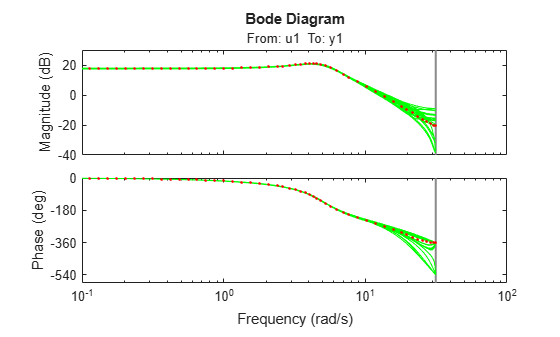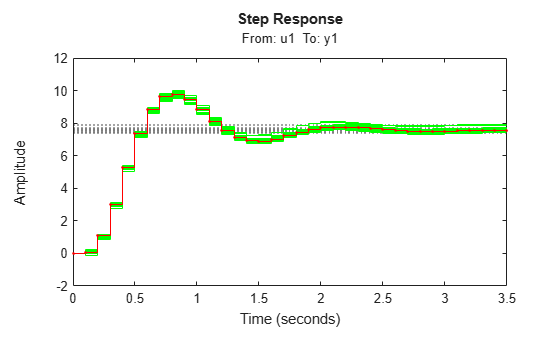# rsample

Random sampling of linear identified systems

## Syntax

```sys_array = rsample(sys,N) sys_array = rsample(sys,N,sd) ```

## Description

```sys_array = rsample(sys,N)``` creates `N` random samples of the identified linear system, `sys`. `sys_array` contains systems with the same structure as `sys`, whose parameters are perturbed about their nominal values, based on the parameter covariance.

```sys_array = rsample(sys,N,sd)``` specifies the standard deviation level, `sd`, for perturbing the parameters of `sys`.

## Input Arguments

 `sys` Identifiable system. `N` Number of samples to be generated. Default: `10` `sd` Standard deviation level for perturbing the identifiable parameters of `sys`. Default: `1`

## Output Arguments

 `sys_array` Array of random samples of `sys`. If `sys` is an array of models, then the size of `sys_array` is equal to ```[size(sys) N]```. There are `N` randomized samples for each model in `sys`. The parameters of the samples in `sys_array` vary from the original identifiable model within 1 standard deviation of their nominal values.

## Examples

collapse all

Estimate a third-order, discrete-time, state-space model.

```load iddata2 z2; sys = n4sid(z2,3);```

Randomly sample the estimated model.

```N = 20; sys_array = rsample(sys,N);```

Analyze the uncertainty in time (step) and frequency (Bode) responses.

```opt = bodeoptions; opt.PhaseMatching = 'on'; figure; bodeplot(sys_array,'g',sys,'r.',opt)``````figure; stepplot(sys_array,'g',sys,'r.-')```Estimate a third-order, discrete-time, state-space model.

```load iddata2 z2; sys = n4sid(z2,3);```

Randomly sample the estimated model. Specify the standard deviation level for perturbing the model parameters.

```N = 20; sd = 2; sys_array = rsample(sys,N,sd);```

Analyze the model uncertainty.

```figure; bode(sys_array);```Estimate an ARMAX model.

```load iddata1 z1 sys = armax(z1,[2 2 2 1]);```

Randomly sample the ARMAX model. Perturb the model parameters up to 2 standard deviations.

```N = 20; sd = 2; sys_array = rsample(sys,N,sd);```

Compare the frequency response confidence region corresponding to 2 standard deviations (asymptotic estimate) with the model array response.

```opt = bodeoptions; opt.PhaseMatching = 'on'; opt.ConfidenceRegionNumberSD = 2; bodeplot(sys_array,'g',sys,'r',opt)```To view the confidence region, right click the plot, and choose Characteristics > Confidence Region.

## Tips

• For systems with large parameter uncertainties, the randomized systems may contain unstable elements. These unstable elements may make it difficult to analyze the properties of the identified system. Execution of analysis commands, such as `step`, `bode`, `sim`, etc., on such systems can produce unreliable results. Instead, use a dedicated Monte-Carlo analysis command, such as `simsd`.

## Version History

Introduced in R2012a